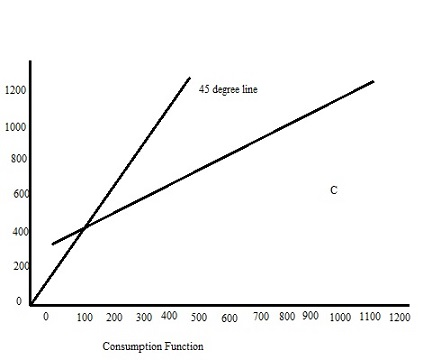# 1. According to the Rule of 72, how many years will it take for GDP to double if the economy is...

## Question:

1. According to the Rule of 72, how many years will it take for GDP to double if the economy is growing at

(a) 1.5 percent a year?

(b) 2.8 percent a year?

2. On a graph, draw the consumption function {eq}C = $200 + 0.75YD {/eq} and the 45 degree line. You may use Excel to do it or just use a ruler to do it manually. (a) At what level of income do households begin to save? Designate that point on the graph with the letter A. (b) By how much does consumption increase when income rises$200 beyond point A? Designate this new level of consumption with point B.

(c) Illustrate the impact on consumption of an increase in consumer confidence.

3. Suppose that furniture production encompasses the following stages:

Stage 1: Trees are sold to lumber company. $8,000 Stage 2: Lumber is sold to furniture company.$17,000

Stage 3: Furniture company sells furniture to retail store. $28,000 Stage 4: Furniture store sells furniture to consumer.$56,000

(a) What is the value added at each stage?

(b) How much does this output contribute to GDP?

(c) How would answer (b) change if the lumber were imported from Canada?

6. Assume the banking system contains the following amounts:

Total reserves $80 billion Transactions deposits$800 billion

Cash held by public $100 billion Reserve requirement 0.10 (a) Are the banks fully utilizing their lending capacity? Explain why. What would happen to the money supply initially if the public deposited another$30 billion of cash in transactions accounts? What would the lending capacity of the banking system be after such a portfolio switch?

(b) How large would the money supply be if the banks fully utilized their lending capacity? What three steps could the Fed take to offset that potential growth in M1?

## Gross Domestic Product:

Gross domestic Product is of different type

1) Real Gross Domestic Product

2) Nominal Domestic Product

3) Gross National Product

4) Net Domestic Product

Gross domestic product calculated through the formula GDP = C+I+G+(X-M) where, C = Consumption, I = Investment, G = Government Investment, (X-M) = Export-Import.

1)

a)

Formula of Rule of 72

r *t = 72

Here,

Net growth rate per period = R

No. of Period = t

Calculation of time period

R*t = 72

t = 72/R

t = 72/1.5

t= 48 Years

Hence,

According to rule 72 for doubling GDP . Net Growth Rate = 48 years

b)

Formula of Rule of 72

r *t = 72

Here,

Net growth rate per period = R

No. of Period = t

Calculation of time period

R*t = 72

t = 72/R

t = 72/2.8

t= 25.71 Years

Hence,

According to rule 72 for doubling GDP . Net Growth Rate = 25.71 years

2)3)

a)

Stage Value of Transaction Added Value
1 8000 $800 2 17000$9,000
3 28000 $11,000 4 56000$28,000

b)

Calculation of contribution of the output to GDP

Contribution of GDP = ($800 + 9000+11000+28000) =$48800

Hence,

Contribution of output to GDP = $48800 C) Calculation of contribution to GDP Contrubution of output to GDP = (9000+11000++28000) =$48000

Hence,

Contribution of output to GDP = $48000 6) a.) Required reserve = 0.10*$800 =$80 billion Excess reserve =$80-$80 = 0 Hence, Bank fully utilise the capacity b) Deposits =$50+$800 =$850 billion

Required reserve =$850 billion * 0.10 =$85 billion

Money supply will increase by =50-5 = \$45 billion